# At the instant shown, cars A and B are traveling at velocities of 58 m/s and...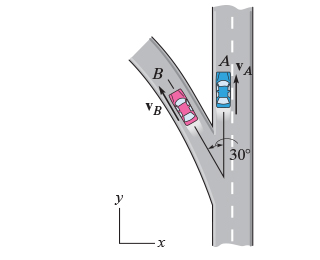At the instant shown, cars A and B are traveling at velocities of 58 m/s and 32 m/s , respectively. B is increasing its velocity by 2 m/s2, while A maintains a constant velocity. The radius of curvature at B is?B = 200 m.

Part A

Determine the magnitude of the velocity of B with respect to A.

Part B

Determine the direction angle of the velocity of B with respect to A.

Part C

Determine the magnitude of the acceleration of B with respect to A.

Part D

Determine the direction angle of the acceleration of B with respect to A.

Concepts and reason

The concepts used to solve this problem are curvilinear motion and relative velocity.

Curvilinear motion:

A particle is said to follow a curvilinear motion if the particle moves on a path other than a straight line or along a curved path.

As in this problem car $B$ moves on a curved path so it has a curvilinear motion.

Velocity in a curvilinear motion:

The direction of velocity of car $B$ , moving along a curved path, is always tangential to the path followed by it. So, the direction of velocity changes at every instant of the motion. The magnitude of velocity also changes at every instant because car $B$ moves with a given acceleration.

Acceleration in curvilinear motion:

Acceleration is defined as rate of change of velocity. But in case of curvilinear motion both the magnitude and direction of velocity vary with time. So, acceleration in this case has two components tangential and normal component of acceleration. The net acceleration is the vector sum of the both the components of acceleration.

Tangential component of acceleration:

Tangential component comes into effect due to change in the magnitude of velocity at every instant. This component always acts tangentially to the path of the particle.

Centripetal acceleration or normal component of acceleration:

Normal component comes into effect due to change in the direction of velocity at every instant. This component always acts towards the center of the curved path of the particle.

Relative velocity:

It is the velocity of one body with respect to the other body, assuming the other body to be at rest. Relative velocity comes handy when the two bodies are in motion and one has to analyze the motion of one of the body with respect to the other.

Vector Quantity:

These are quantities that require magnitude and direction both to describe them completely. A few examples are velocity, force, acceleration and energy.

Vector Resolution

This is a technique to divide a vector into rectangular components. For the purpose of this problem the vector is divided into two components. The component of a vector along the x-axis is called horizontal component and the component of a vector along the y-axis is called vertical component.

Initially, find the velocity vector of car $A$ and car $B$ . Then, determine the relative velocity in vector form. Use expression for magnitude of a vector to determine the relative velocity of car $B$ with respect to car $A$ . Then, find the acceleration vector of car $A$ and car $B$ . Finally, determine the relative acceleration in form of vector. Use expression for magnitude of a vector to determine the relative acceleration of car $B$ with respect to car $A$ .

Fundamentals

Representation of vector in Cartesian form:

A vector is represented in Cartesian form as,

$\vec A = \left( {x{\bf{\hat i}} + y{\bf{\hat j}}} \right)$

Here, $x$ and $y$ are the components of the vector on positive x axis and positive y axis respectively and ${\bf{\hat i}}$ , ${\bf{\hat j}}$ are the unit vectors along x axis and y axis respectively.

Magnitude of $\mathop A\limits^ \to$ is,

$\left| {\vec A} \right| = \sqrt {{x^2} + {y^2}}$

The expression for direction of vector $\vec A$ is written as,

$\varphi = {\tan ^{ - 1}}\left( {\frac{y}{x}} \right)$

Here, $\varphi$ is the direction of $\vec A$ with respect to positive x axis.

Vector resolution:

For a vector $V$ making an angle $\theta$ with the positive x axis, the vector resolution to obtain the rectangular components is shown as,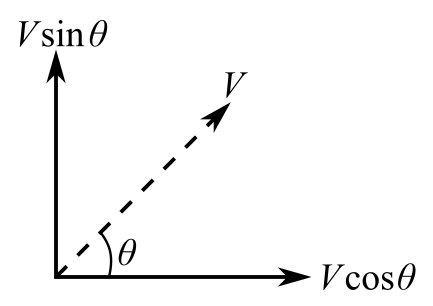The horizontal component along positive x axis $\left( {{V_x}} \right)$ and vertical component along positive y axis $\left( {{V_y}} \right)$ is,

${V_x} = V\cos \theta$

${V_y} = V\sin \theta$

Sign Convention:

The component along positive direction of x axis is taken as positive and vice versa.

The component along positive direction of y axis is taken as positive and vice versa.

Relative velocity of car $B$ with respect to car $A$ is,

${\vec v_{BA}} = {\vec v_B} - {\vec v_A}$

Here, ${\vec v_{BA}}$ is the relative velocity of car $B$ with respect to car $A$ , ${\vec v_A}$ is the velocity of car $A$ and ${\vec v_B}$ is the velocity of car $B$ .

Relative acceleration of car $B$ with respect to car $A$ is,

${\vec a_{BA}} = {\vec a_B} - {\vec a_A}$

Here, ${\vec a_{BA}}$ is the relative acceleration of car $B$ with respect to car $A$ , ${\vec a_A}$ is the acceleration of car $A$ and ${\vec a_B}$ is the acceleration of car $B$ .

Expression for Centripetal acceleration is,

${\left( {{a_B}} \right)_c} = \frac{{{v_B}^2}}{R}$

Here, ${\left( {{a_B}} \right)_c}$ is the centripetal acceleration of the car $B$ , ${v_B}$ is the speed of car $B$ and $R$ is the radius of the curved path.

(A)

Draw the vector diagram of the given situation for both the cars.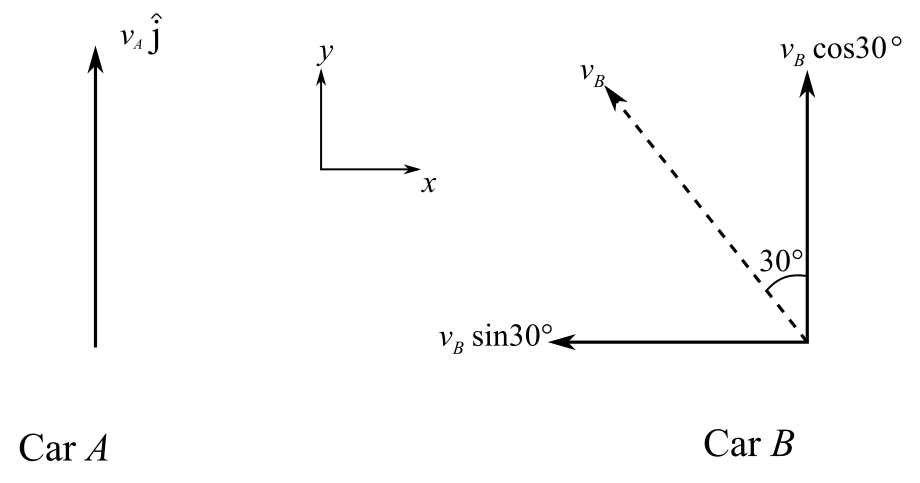Calculate the velocity of car A as,

${{\bf{\vec v}}_{\bf{A}}} = {v_A}{\rm{ }}{\bf{\hat j}}$

Substitute $\left( {{\rm{58 m/s}}} \right)$ for ${v_A}$ .

${{\bf{\vec v}}_{\bf{A}}} = \left( {{\rm{58 m/s}}} \right){\rm{ }}{\bf{\hat j}}$

Calculate the velocity of car B as,

${{\bf{\vec v}}_B} = - \left( {{v_B}\sin 30^\circ } \right){\rm{ }}{\bf{\hat i}} + \left( {{v_B}\cos 30^\circ } \right){\rm{ }}{\bf{\hat j}}$

Substitute $\left( {{\rm{32 m/s}}} \right)$ for ${v_B}$ .

${{\bf{\vec v}}_B} = - \left( {{\rm{16 m/s}}} \right){\rm{ }}{\bf{\hat i}} + \left( {{\rm{27}}{\rm{.7 m/s}}} \right){\rm{ }}{\bf{\hat j}}$

Write the expression for relative velocity of car B with respect to car A.

${{\bf{\vec v}}_{{\bf{BA}}}} = {{\bf{\vec v}}_{\bf{B}}} - {{\bf{\vec v}}_{\bf{A}}}$

Substitute $\left( {{\rm{58 m/s}}} \right){\rm{ }}{\bf{\hat j}}$ for ${{\bf{\vec v}}_{\bf{A}}}$ and $- \left( {{\rm{16 m/s}}} \right){\rm{ }}{\bf{\hat i}} + \left( {{\rm{27}}{\rm{.7 m/s}}} \right){\rm{ }}{\bf{\hat j}}$ for ${{\bf{\vec v}}_B}$ in the above expression of the relative speed expression,

$\begin{array}{c}\\{{{\bf{\vec v}}}_{{\bf{BA}}}} = - \left( {{\rm{16 m/s}}} \right){\rm{ }}{\bf{\hat i}} + \left( {{\rm{27}}{\rm{.7 m/s}}} \right){\rm{ }}{\bf{\hat j}} - \left( {{\rm{58 m/s}}} \right){\rm{ }}{\bf{\hat j}}\\\\ = - \left( {{\rm{16 m/s}}} \right){\rm{ }}{\bf{\hat i}} + \left( { - 30.3{\rm{ m/s}}} \right){\rm{ }}{\bf{\hat j}}\\\\ = \left( { - 16{\rm{ }}{\bf{\hat i}} - 30.3{\rm{ }}{\bf{\hat j}}} \right){\rm{ m/s}}\\\end{array}$

Obtain the expression of the magnitude of the velocity ${{\bf{\vec v}}_{{\bf{BA}}}}$ as,

$\left| {{{{\bf{\vec v}}}_{{\bf{BA}}}}} \right| = \sqrt {\left( {{{{\bf{\vec v}}}_{{\bf{BA}}}}} \right)_x^2 + \left( {{{{\bf{\vec v}}}_{{\bf{BA}}}}} \right)_y^2}$

Substitute $- 16{\rm{ m/s}}$ for ${\left( {{{{\bf{\vec v}}}_{{\bf{BA}}}}} \right)_x}$ and $- 30.3\,{\rm{m/s}}$ for ${\left( {{{{\bf{\vec v}}}_{{\bf{BA}}}}} \right)_y}$ .

$\begin{array}{c}\\\left| {{{{\bf{\vec v}}}_{{\bf{BA}}}}} \right| = \sqrt {{{\left( { - 16} \right)}^2} + {{\left( { - 30.3} \right)}^2}} {\rm{ m/s}}\\\\ = 34.3{\rm{ m/s}}\\\end{array}$

(B)

Obtain the expression for direction for the relative velocity of car $B$ with respect to x axis as,

$\varphi = {\tan ^{ - 1}}\left( {\frac{{{{\left( {{{{\bf{\vec v}}}_{{\bf{BA}}}}} \right)}_y}}}{{{{\left( {{{{\bf{\vec v}}}_{{\bf{BA}}}}} \right)}_x}}}} \right)$

Here, $\varphi$ is the direction angle of velocity for the relative velocity of car B, ${\left( {{{{\bf{\vec v}}}_{{\bf{BA}}}}} \right)_x}$ and ${\left( {{{{\bf{\vec v}}}_{{\bf{BA}}}}} \right)_y}$ are the x and y component of ${{\rm{\vec a}}_{{\rm{AB}}}}$ .

Substitute $- 16{\rm{ m/s}}$ for ${\left( {{{{\bf{\vec v}}}_{{\bf{BA}}}}} \right)_x}$ and $- 30.3\,{\rm{m/s}}$ for ${\left( {{{{\bf{\vec v}}}_{{\bf{BA}}}}} \right)_y}$ in the above expression of the direction of velocity vector.

$\varphi = {\tan ^{ - 1}}\left( {\frac{{ - 30.3{\rm{ m/s}}}}{{ - 16{\rm{ m/s}}}}} \right)$

Since both the x and y components of ${{\bf{\vec v}}_{{\bf{BA}}}}$ are negative the angle $\varphi$ must lie in the third quadrant. This implies that,

$\varphi = 242.2^\circ$

(C)

Draw the vector diagram for acceleration of car B.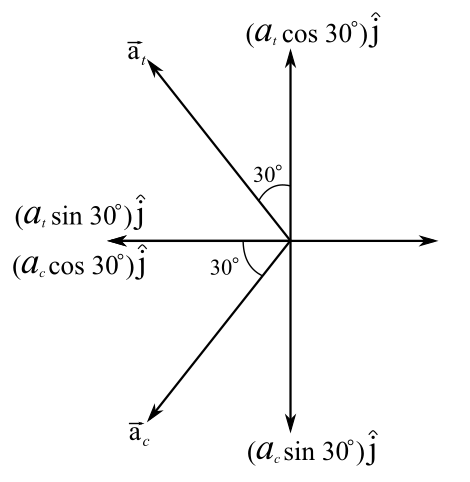Acceleration of car A is zero as the velocity is constant.

Acceleration of car B is given as follows:

$\begin{array}{c}\\{{{\bf{\vec a}}}_{\bf{B}}} = - \left( {{a_t}\sin 30^\circ + {a_c}\cos 30^\circ } \right){\bf{\hat i}} + \left( {{a_t}\cos 30^\circ - {a_c}\sin 30^\circ } \right){\bf{\hat j}}\\\\ = - \left( {{a_t}\sin 30^\circ + \frac{{{v_B}^2}}{R}\cos 30^\circ } \right){\rm{ }}{\bf{\hat i}} + \left( {{a_t}\cos 30^\circ - \frac{{{v_B}^2}}{R}\sin 30^\circ } \right){\rm{ }}{\bf{\hat j}}\\\\ = - \left( {2\sin 30^\circ + \frac{{{{32}^2}}}{{200}}\cos 30^\circ } \right){\rm{ }}{\bf{\hat i}} + \left( {2\cos 30^\circ - \frac{{{{32}^2}}}{{200}}\sin 30^\circ } \right){\rm{ }}{\bf{\hat j}}\\\\ = \left( { - 5.434{\rm{ }}{\bf{\hat i}} - 0.828{\rm{ }}{\bf{\hat j}}} \right){\rm{ m/}}{{\rm{s}}^2}\\\end{array}$

Obtain the expression for relative acceleration of car B to that of car A as,

${{\bf{\vec a}}_{{\bf{BA}}}} = {{\bf{\vec a}}_{\bf{B}}} - {{\bf{\vec a}}_{\bf{A}}}$

Substitute $\left( { - 5.434{\rm{ }}{\bf{\hat i}} - 0.828{\rm{ }}{\bf{\hat j}}} \right){\rm{ m/}}{{\rm{s}}^2}$ for ${{\bf{\vec a}}_{\bf{B}}}$ and $0$ for ${{\bf{\vec a}}_{\bf{A}}}$ .

$\begin{array}{c}\\{{{\bf{\vec a}}}_{{\bf{BA}}}} = \left( { - 5.434{\rm{ }}{\bf{\hat i}} - 0.828{\rm{ }}{\bf{\hat j}}} \right){\rm{ m/}}{{\rm{s}}^2} - 0{\rm{ m/}}{{\rm{s}}^2}\\\\ = \left( { - 5.434{\rm{ }}{\bf{\hat i}} - 0.828{\rm{ }}{\bf{\hat j}}} \right){\rm{ m/}}{{\rm{s}}^2}\\\end{array}$

From the magnitude expression the expression of the magnitude of the relative acceleration of car B is expresses as follows,

$\begin{array}{c}\\\left| {{{{\bf{\vec a}}}_{{\bf{BA}}}}} \right| = \sqrt {{{\left( { - 5.434} \right)}^2} + {{\left( { - 0.828} \right)}^2}} {\rm{ m/}}{{\rm{s}}^2}\\\\ = 5.497{\rm{ m/}}{{\rm{s}}^2}\\\\ \simeq 5.5{\rm{ m/}}{{\rm{s}}^2}\\\end{array}$

(D)

Write the expression of the direction angle for the relative acceleration of car $B$ with respect to x axis as,

$\alpha = {\tan ^{ - 1}}\left( {\frac{{{{\left( {{{{\bf{\vec a}}}_{{\bf{BA}}}}} \right)}_y}}}{{{{\left( {{{{\bf{\vec a}}}_{{\bf{BA}}}}} \right)}_x}}}} \right)$

Here, $\alpha$ is the direction acceleration for the relative acceleration of car B, ${\left( {{{{\bf{\vec a}}}_{{\bf{BA}}}}} \right)_{\bf{x}}}$ and ${\left( {{{{\bf{\vec a}}}_{{\bf{BA}}}}} \right)_{\bf{y}}}$ are the x and y component of ${{\bf{\vec a}}_{{\bf{AB}}}}$ .

Substitute $- 0.828{\rm{ m/}}{{\rm{s}}^2}$ for ${\left( {{{{\bf{\vec a}}}_{{\bf{BA}}}}} \right)_{\bf{y}}}$ and $- 5.434{\rm{ m/}}{{\rm{s}}^2}$ for ${\left( {{{{\bf{\vec a}}}_{{\bf{BA}}}}} \right)_{\bf{x}}}$ .

$\alpha = {\tan ^{ - 1}}\left( {\frac{{ - 0.828{\rm{ m/}}{{\rm{s}}^2}}}{{ - 5.434{\rm{ m/}}{{\rm{s}}^2}}}} \right)$

Since both the x and y components of ${{\bf{\vec a}}_{{\bf{BA}}}}$ are negative the angle $\alpha$ must lie in the third quadrant. This implies that,

$\alpha = 188.67^\circ$

Ans: Part A

The magnitude of velocity B with respect to A is equal to $34.3{\rm{ m/s}}$ .

Part B

The direction angle of the velocity of $B$ with respect to $A$ is equal to $242.2^\circ$ .

Part C

The magnitude of acceleration of $B$ with respect to $A$ is equal to $5.5{\rm{ m/}}{{\rm{s}}^2}$ .

Part D

The direction angle of the acceleration component of $B$ with respect to $A$ is equal to $188.67^\circ$ .

#### Earn Coin

Coins can be redeemed for fabulous gifts.

Similar Homework Help Questions
• ### At the instant shown, cars A and B are traveling at velocities of 50 m/s and...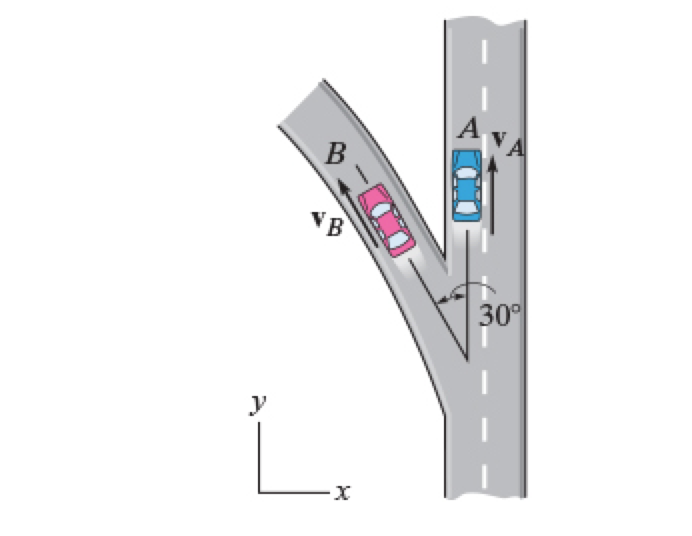At the instant shown, cars A and B are traveling at velocities of 50 m/s and 30 m/s , respectively. B is increasing its velocity by 2 m/s2, while A maintains a constant velocity. The radius of curvature at B is ?B = 200 m. Determine the magnitude of the velocity of B with respect to A. Determine the direction angle of the velocity of B with respect to A. Determine the magnitude of the acceleration of B with respect...

• ### At the instant shown, cars A and B are traveling at the speeds v A =...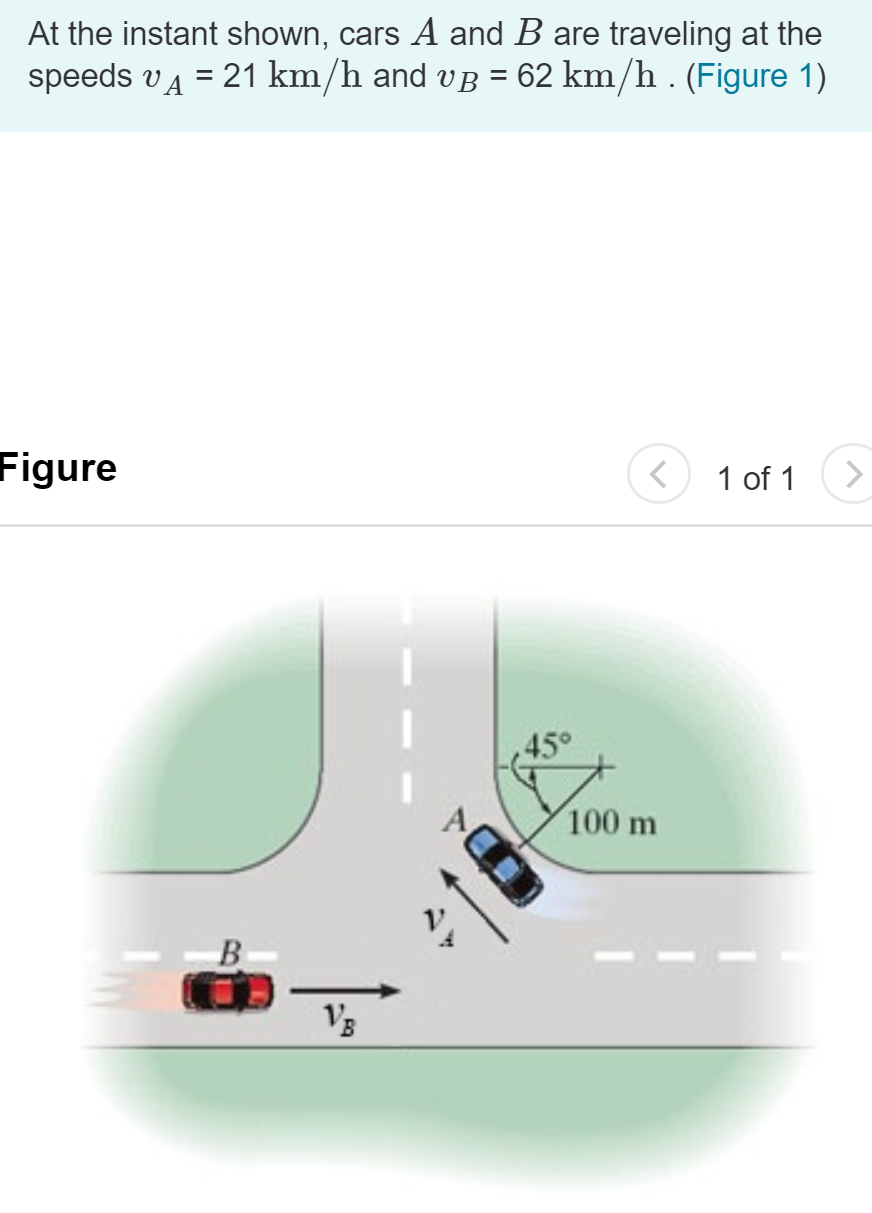At the instant shown, cars A and B are traveling at the speeds v A = 21 km/h and vB = 62 km/h . (Figure 1) Figure 1 of 1 100 m Part A If B is accelerating at 1210 km/h? while A maintains a constant speed, determine the velocity of A with respect to B. Express your answer to three significant figures and include the appropriate units. I MÅR O 2 ? V A/B = 78.27 Submit Request Answer...

• ### Need MATLAB code to solve the following: At the instant shown cars A and B are...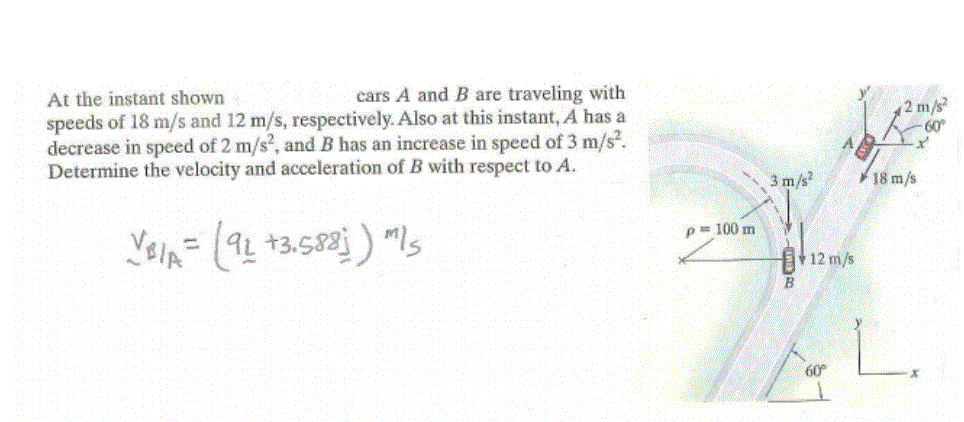Need MATLAB code to solve the following: At the instant shown cars A and B are traveling Need to solve using MATLAB Would like the code / syntax to solve with MATLAB cars A and B are traveling with At the instant shown speeds of 18 m/s and 12 m/s, respectively. Also at this instant, A has a decrease in speed of 2 m/s2, and B has an increase in speed of 3 m/s Determine the velocity and acceleration of...

• ### A particle is traveling counterclockwise in a circle of radius r= 2.40 m. At some instant...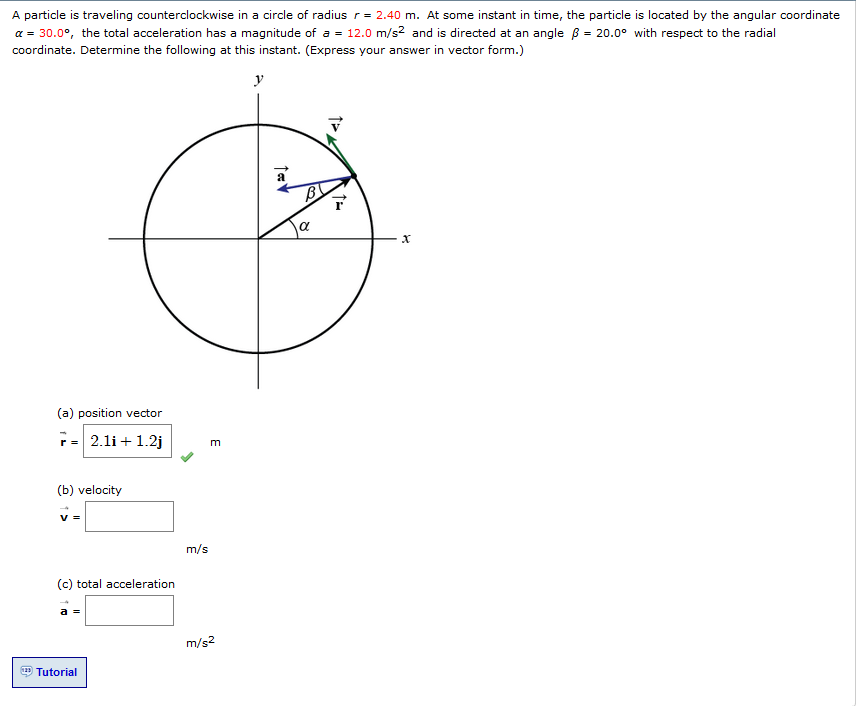A particle is traveling counterclockwise in a circle of radius r= 2.40 m. At some instant in time, the particle is located by the angular coordinate α-30.0°, the total acceleration has a magnitude of a-12.0 m/s2 and is directed at an angle β-20.0o with respect to the radial coordinate. Determine the following at this instant. (Express your answer in vector form.) (a) position vector H 2.11+ 12] (b) velocity 哭: Infss (c) total acceleration Tutorial

• ### 2) At the instant shown, cars A and B are traveling at the speeds shown. Determine...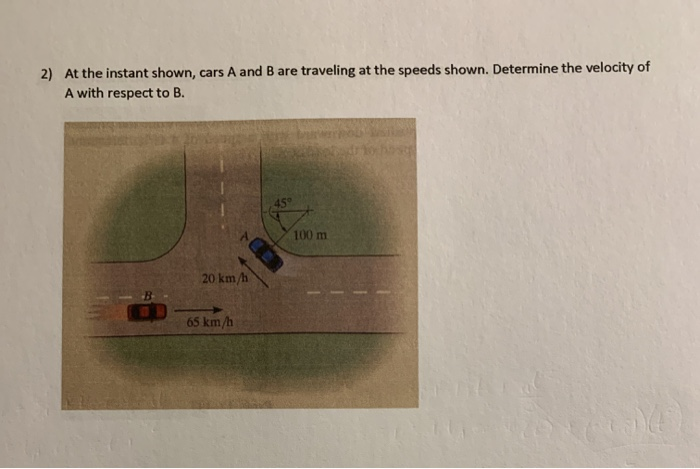2) At the instant shown, cars A and B are traveling at the speeds shown. Determine the velocity of A with respect to B. 100 m 20 km/h 65 km/h

• ### Dynamics Help

At the instant shown, cars A and B are traveling atspeeds of 55mi/h and 40mi/h, respectively. If B is increasing its speed by 1200 mi/h2, while A maintains a constant speed, determine the velocity and accelerationof B with respect to A. Car B moves along the curve having a radius of curvature of 0.5 mi.

• ### MAGNITUDE AND DIRECTION OF ACCELERATION

At the instant shown, cars and travel at speeds of 70 and 50 , respectively.A)If is decreasing its speed at 1400 while is increasing its speed at800 ,determine the direction of the the acceleration of with respectto . Car moves along a curve having a radius of curvature of 0.7 .B)Determine the magnitude of the the acceleration of with respect to .

• ### 1) At the instant shown, car A is traveling East with a velocity of 25 m/s...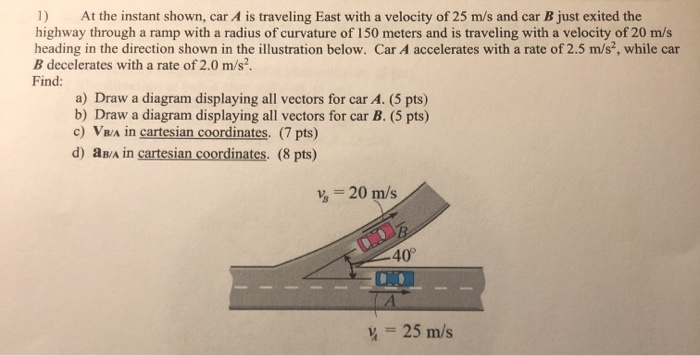1) At the instant shown, car A is traveling East with a velocity of 25 m/s and car B just exited the highway through a ramp with a radius of curvature of 150 meters and is traveling with a velocity of 20 m/s heading in the direction shown in the illustration below. Car A accelerates with a rate of 2.5 m/s, while car B decelerates with a rate of 2.0 m/s. Find: a) Draw a diagram displaying all vectors for...

• ### At the instant shown collar B moves with a velocity of vB=4 [m/s] and acceleration aB=2.4...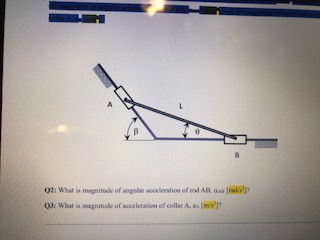At the instant shown collar B moves with a velocity of vB=4 [m/s] and acceleration aB=2.4 [m/s2 ] to the right. Angle =50o , and length of the rod is L=650 [mm]. At the instant when =30o , determine (a) magnitude of angular acceleration of rod AB, AB [rad/s2 ], and (b) magnitude of acceleration of collar A, aA [m/s2 ]. What is magnitude of angular acceleration of rod AB, AB [rad/s2 ]? What is magnitude of acceleration of collar...